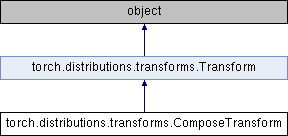Caffe2 - Python API A deep learning, cross platform ML framework
torch.distributions.transforms.ComposeTransform Class Reference
Inheritance diagram for torch.distributions.transforms.ComposeTransform:## Public Member Functions

def __init__ (self, parts)

def __eq__ (self, other)

def domain (self)

def codomain (self)

def bijective (self)

def sign (self)

def event_dim (self)

def inv (self)

def __call__ (self, x)

def log_abs_det_jacobian (self, x, y)

def __repr__ (self)Public Member Functions inherited from torch.distributions.transforms.Transform
def __init__ (self, cache_size=0)

def inv (self)

def sign (self)

def __eq__ (self, other)

def __ne__ (self, other)

def __call__ (self, x)

def log_abs_det_jacobian (self, x, y)

def __repr__ (self)

## Public Attributes

partsStatic Public Attributes inherited from torch.distributions.transforms.Transform
bijective

event_dim

## Detailed Description

```Composes multiple transforms in a chain.
The transforms being composed are responsible for caching.

Args:
parts (list of :class:`Transform`): A list of transforms to compose.
```

Definition at line 210 of file transforms.py.

The documentation for this class was generated from the following file: